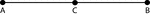### Segment, 3 Points on a Line

Illustration of line segment AB, which is made up of line segments AC and CB. C is considered the midpoint…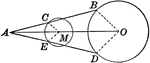### Midpoint of Straight Line Drawn From an Exterior point to Circumference

Illustration showing a line with a midpoint drawn from a given exterior point to a given circumference.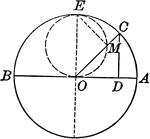### Circle Made by Ends of a Line Touching Two Fixed Lines Perpendicular to Each Other

Illustration showing a line that moves so that its ends constantly touch two fixed lines which are perpendicular…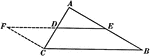### Line Joining Midpoints on a Triangle

Illustration used to prove "The line joining the mid-points of two sides of a triangle is parallel to…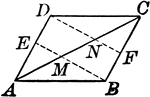### Parallelogram With Lines From opposite Vertices to Midpoints

Illustration to show that when lines from two opposite vertices of a parallelogram to the midpoints…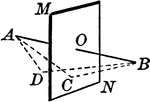### Locus Point to a Plane

"The locus of a point in space equidistant from the extremities of a straight line is the plane perpendicular…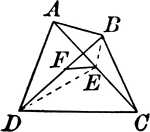### Quadrilateral With Diagonals and Midpoints Joined

Quadrilateral with intersecting diagonals and segment connecting midpoints.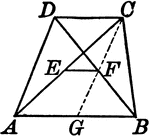### Trapezoid With Lines Joining the Midpoints of the Diagonals

Illustrations of a trapezoid with a line joining the midpoints of the diagonals.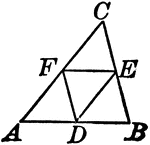### Midpoints of Triangle Divide Triangle into Four Equal Triangles

Illustration to show that if the lines joining the middle points of the sides of a triangle divide the…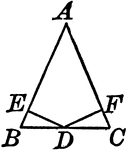### Perpendiculars Dropped From Midpoint of Base to Legs of Isosceles Triangles

Illustration to show the perpendiculars dropped from the midpoint of the base to the legs of an isosceles…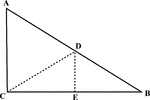### Right Triangle With Midpoint of Hypotenuse Drawn

Illustration of a right triangle with the midpoint of the hypotenuse drawn.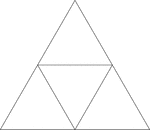### Equilateral Triangle Inscribed In An Equilateral Triangle

Illustration of an equilateral triangle inscribed in an equilateral triangle. The smaller triangle is…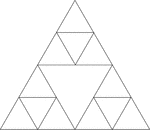### Equilateral Triangles Inscribed In An Equilateral Triangle

Illustration of an equilateral triangle inscribed in an equilateral triangle by joining the midpoints…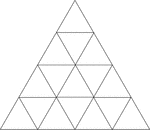### Equilateral Triangles Inscribed In Equilateral Triangles

Illustration of an equilateral triangle inscribed in an equilateral triangle by joining the midpoints…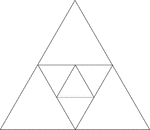### Equilateral Triangles Inscribed In Equilateral Triangles

Illustration of an equilateral triangle inscribed in an equilateral triangle by joining the midpoints…